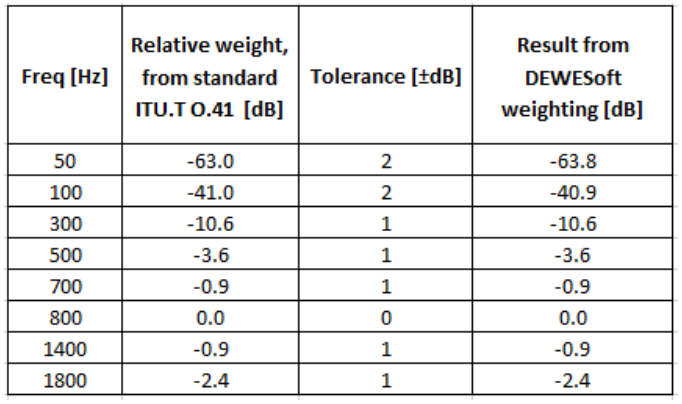# Psophometer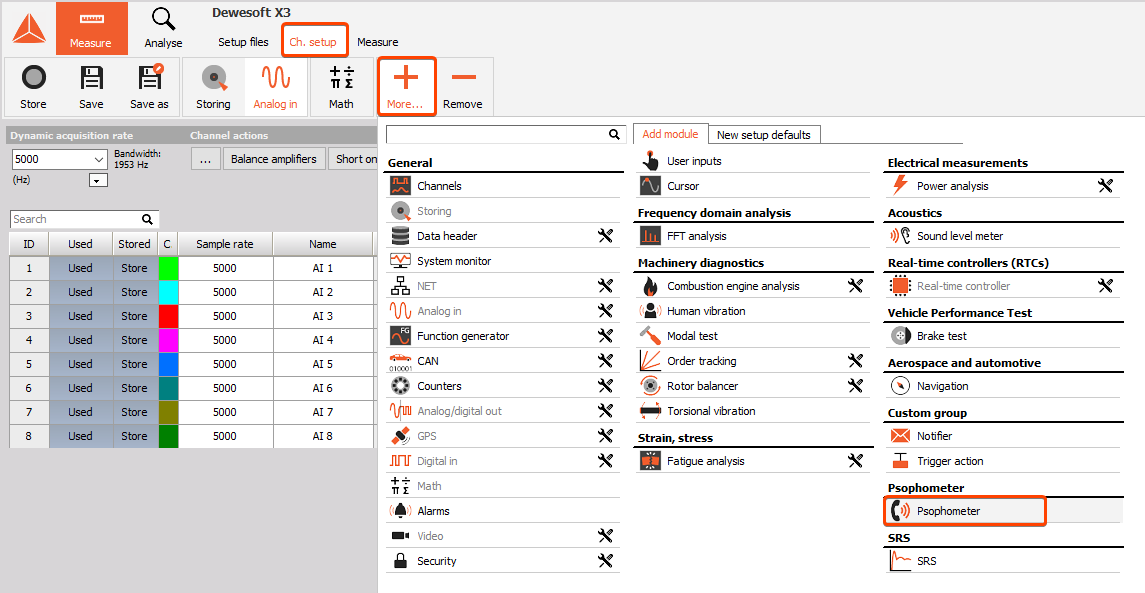When the plugin is added, you will see the icon in the channel setup menu. And after selecting the plugin, you have to choose the input channels and the psophometer settings.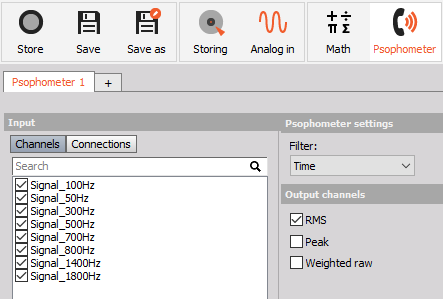• Frequency filter: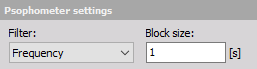Block size is calculated from the defined block size [s] and the sample rate.

For example, if we have a sample rate of 20 kHz and we define the block size as 1 s. First, we multiply these two numbers to get the block size number in terms of samples, so $20 000 s^{-1} * 1 s = 20 000 samples$. Since the psophometer uses an FFT, the number of samples needs to be defined as $2^n$ (n is any integer). Since no n in the equation $2^n$ produces the number of sample which would match our block size (20 000 samples), DEWESoft simply takes the closest number of samples from the equation. In this case, $2^{15} = 32768$ is the nearest largest block size. DEWESoft then converts the samples into a time block, this would equal $\large\frac {32768 samples} {20000 s^{-1}} = 1.6384$ second time block.

If you want to use an exact block size, then you have to adjust your sample rate so that it matches a possible FFT rate. Also, there is no overlap in the calculations, every block is calculated on its own.

The frequency weighting curve in DEWESoft is compliant with the table in standard ITU-T O.41.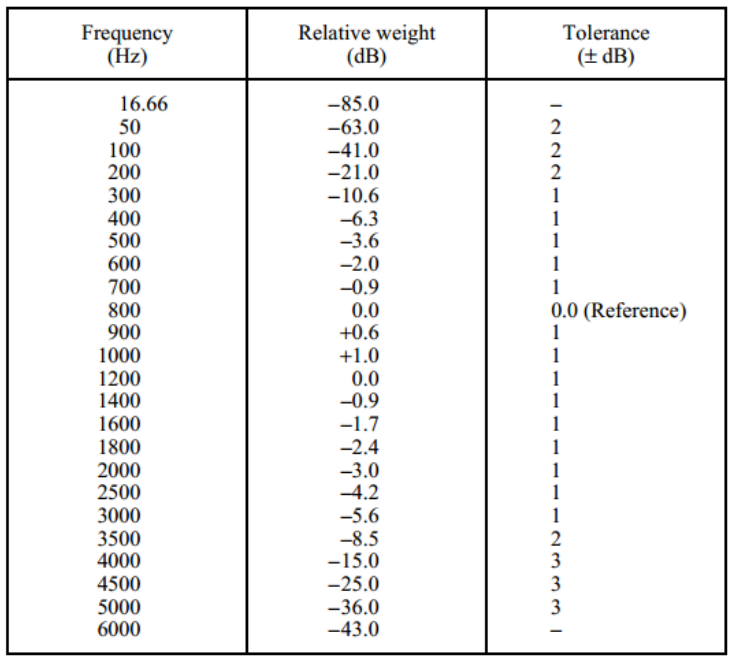• Time filter: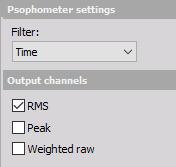Time filter outputs the same result as the frequency filter, but in another way. Frequency filter applies weighting coefficients to each frequency. Time filter applies a filter on the time domain data that has the same characteristics as the frequency weighting curve.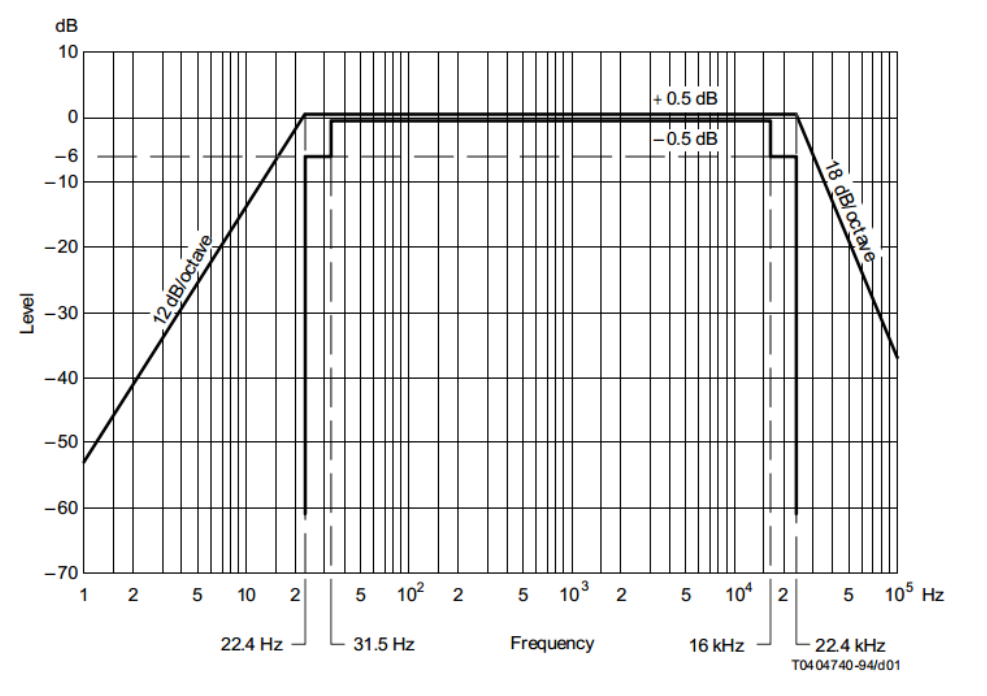The output channels can be selected from RMS, peak or weighted raw.

Name Description
RMS RMS of the weighted signal
Peak Peak of the weighted signal
Weighted raw Weighted raw time signal

## Results in DEWESoft

To check the compatibility with the ITU-T O.41 international telecommunication standard we made a simple test with psophometer plugin in DEWESoft to see the frequency weightings.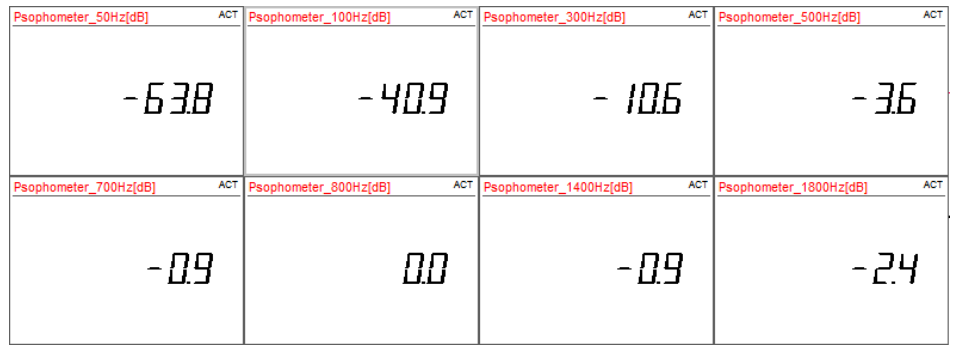Comparison of the results from DEWESoft with the standard ITU-T O.41.